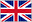# week 3 UML

0    26 fiche

questionréponsewhat does UML stand for?
Unified Modeling Language
what does UML do?
Provides notation in the form of diagrams for modeling and documenting a broad range of systems, activities, and processes.
what is a model
can be considered as an abstraction of a system (problem), that consists of objects interacting by sending messages
What is a domain
The domain is the actual world from which the system (problem) originates.
Generally, more than one model is used to describe complex systems. T/F?
True
What is a view?
A view depicts selected aspects of a model in order to visualize and make them understandable
The essential characteristics (data) of the entities are captured as _______(_______), and the essential functionalities (operations, actions, behaviour) of those attributes are captured as _____.
The essential characteristics (data) of the entities are captured as attributes (data fields), and the essential functionalities (operations, actions, behaviour) of those attributes are captured as methods.
What a re the 3 different types of models?
Functional, object, dynamic
What are the functional models?
use case diagrams (actors and use cases)
what are the object models?
class and instance diagrams, which comprise objects (attributes and functionality) and associations (relationships), used to describe the system structure;
what are the dynamic models?
represent a set of operations that describe system’s behaviour.
What is the purpose of a usecase diagram?
to represent functionality of a system during the elicitation and analysis stages (what rather then how)
What is a scenario?
a scenario is a sequence of steps describing what happens when someone interacts with the system
what is an Actor?
the roles that people (objects) play in the system; different type of users (or systems) that interact with the system.
What is association in relation to class diagrams?
a relationship between instances of the two classes, shown as a solid line between the classes (could be directed).
What is aggregation in relation to class diagrams?
a part-of relationship, in which one class belongs to a collection. Shown as solid line with a diamond end pointing to the part containing the whole.
What is generalizationin relation to class diagrams?
an inheritance relationship, shown as a solid line with a triangle pointing to the superclass
multiplicity of association, what does * or 0...* mean?
no upper limit – zero or more
multiplicity of association, what does 1 or 1...1 mean?
exactly one
multiplicity of association, what does 0...1 mean?
zero or one
multiplicity of association, what does 1...* mean?
at least one
what are Interaction Diagrams?
Formalized description and notation of dynamic behaviour and collaboration of group of objects
interaction diagrams what are objects involved called?
participating objects
give 1 example of an interaction diagram
Sequence diagrams
what is a state chart diagram?
The dynamic of a system can be described with a number of states and the transitions between them
What is a state?
A state defines a particular set of attributes (data, characteristics) for an object.